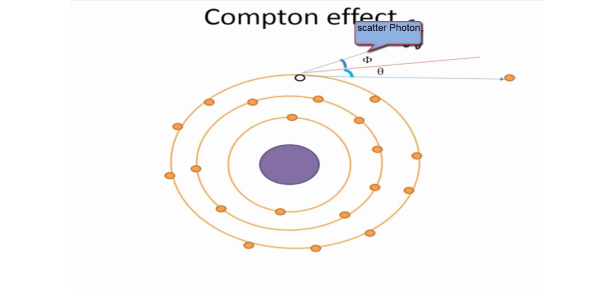# Physics Test: Practice Questions On Compton Effect!

15 Questions | Total Attempts: 2168Settings.

• 1.
The Compton shift Δλ is twice the Compton wavelength if the scattering angle is
• A.

90°

• B.

180°

• C.

45°

• D.

• 2.
According to Rayleigh-Jeans' radiation law, the emissive power of a blackbody over a wavelength range λ to λ+dλ is proportional to λⁿ. Then n is
• A.

4

• B.

1

• C.

-2

• D.

-4

• 3.
The rest mass of a photon of frequency ν is
• A.

Hν/c

• B.

Hν/c²

• C.

Zero

• D.

Hν²/c

• 4.
What is the speed of a particle whose mass is 3 times its rest mass?
• A.

2c/3

• B.

C/3

• C.

√2c

• D.

2√2c/3

• 5.
In Compton scattering, if the angle of scattering varies from 0º to 180º, the electron's recoil angle varies from
• A.

0º to 90º

• B.

90º to 180º

• C.

0º to 180º

• D.

0º to 45º

• 6.
The radiated intensity per unit time from a cavity kept at temperature 1000 Kelvin is 5.67x10ⁿ W/m². The value of n is
• A.

8

• B.

6

• C.

4

• D.

12

• 7.
The de Broglie wavelength of an electron accelerated by a potential of 150 V is
• A.

0.08 Å

• B.

1 Å

• C.

12.26 Å

• D.

150 Å

• 8.
The momentum of an electron (rest mass energy = 0.5 MeV) is 1 MeV/c. Its energy in MeV is
• A.

1.5

• B.

1.12

• C.

1.22

• D.

2.22

• 9.
The de Broglie wavelength of a thermal neutron of mass m at temperature T is given by λ =
• A.

H/√(3mkT)

• B.

H/√(2mkT)

• C.

√3h/(mkT)

• D.

3h/√(2mkT)

• 10.
In Davisson-Germer's experiment the highest peak was obtained at an angle θ = 50° with a voltage of 54 V. The de Broglie wavelength of electrons was
• A.

1.67 Å

• B.

0.167 Å

• C.

0.227 Å

• D.

2.27 Å

• 11.
A proton accelerated through a potential difference of V has the same de Broglie wavelength as an α-particle subjected to a potential difference of
• A.

4V

• B.

V/4

• C.

V/8

• D.

8V

• 12.
GaP, a semiconductor with an energy gap of 2.25 eV, is used to make LEDs. The wavelength of emitted light from pure GaP is
• A.

705 nm

• B.

515 nm

• C.

630 nm

• D.

555 nm

• 13.
The peak wavelengths of radiation of a red-hot and a yellow-hot object are 630 nm and 570 nm respectively. If the red-hot object's temperature is 5000 K, the other's temperature is
• A.

4000 K

• B.

4500 K

• C.

5000 K

• D.

5500 K

• 14.
In relation to Rayleigh-Jeans law, the ultraviolet catastrophe refers to the prediction
• A.

E(ν) → 0 as ν → 0

• B.

E(T) → ∞ as T → 0

• C.

E(λ) → ∞ as λ → 0

• D.

E(λ) → ∞ as λ → ∞

• 15.
According to Planck, the average energy of cavity oscillators in the frequency range ν to ν+dν is given by
• A.

Hν/(exp[hν/kT] - 1)

• B.

Hν/(exp[hν/kT] + 1)

• C.

• D.

Hν.exp[-hν/kT]

• 16.
The energy of photons contained in visible light of wavelength 600 nm is
• A.

2 eV

• B.

2 keV

• C.

2 MeV

• D.

0.02 eV

• 17.
If visible light is used in Compton scattering the Compton shift will be
• A.

Negative

• B.

Zero

• C.

More positive than what is observed with X-rays

• D.

Positive but not detectable in the visible window

• 18.
The number of oscillation modes per unit volume for em standing waves of frequency ν in a blackbody cavity is proportional to
• A.

ν³

• B.

ν²

• C.

ν

• D.

Hν/(exp[hν/kT] - 1)

• 19.
The Compton shift Δλ is half the Compton wavelength if the scattering angle is
• A.

45°

• B.

90°

• C.

60°

• D.

180°

• 20.
Compton scattering is performed on blocks of carbon and silver. If I and I' are the intensities of the unmodified (λ) and Compton-shifted (λ') lines in the scattered X-rays, then
• A.

I > I' for both carbon and silver

• B.

I = I' for both carbon and silver

• C.

I > I' for carbon, I < I' for silver

• D.

I < I' for carbon, I > I' for silver

• 21.
For cavity radiation of wavelength λ at absolute temperature T, Wien's radiation law corresponds to Planck's law when
• A.

Photon's energy

• B.

Photon's energy >> kT

• C.

Photon's energy = kT

• D.

Photon's energy has any finite value

• 22.
If one measures the energy of a photon accurately the uncertainty in the measurement of frequency becomes
• A.

Zero

• B.

• C.

1

• D.

½

• 23.
If the measured momentum of an electron is 3.20 x10ˉ²⁷ kg-m/s with an uncertainty of 1.6 x10ˉ²⁹ kg-m/s, what is the minimum uncertainty in the determination of its position?
• A.

1.3 nanometer

• B.

1.3 micrometer

• C.

3.3 nanometer

• D.

3.3 micrometer

• 24.
The Compton wavelength for electron is
• A.

0.24 Å

• B.

0.024 Å

• C.

2.4 Å

• D.

0.0024 Å

• 25.
The disagreement between Rayleigh-Jeans' predictions and experimental results for blackbody radiation spectrum is known as
• A.

Infrared signature

• B.Back to top